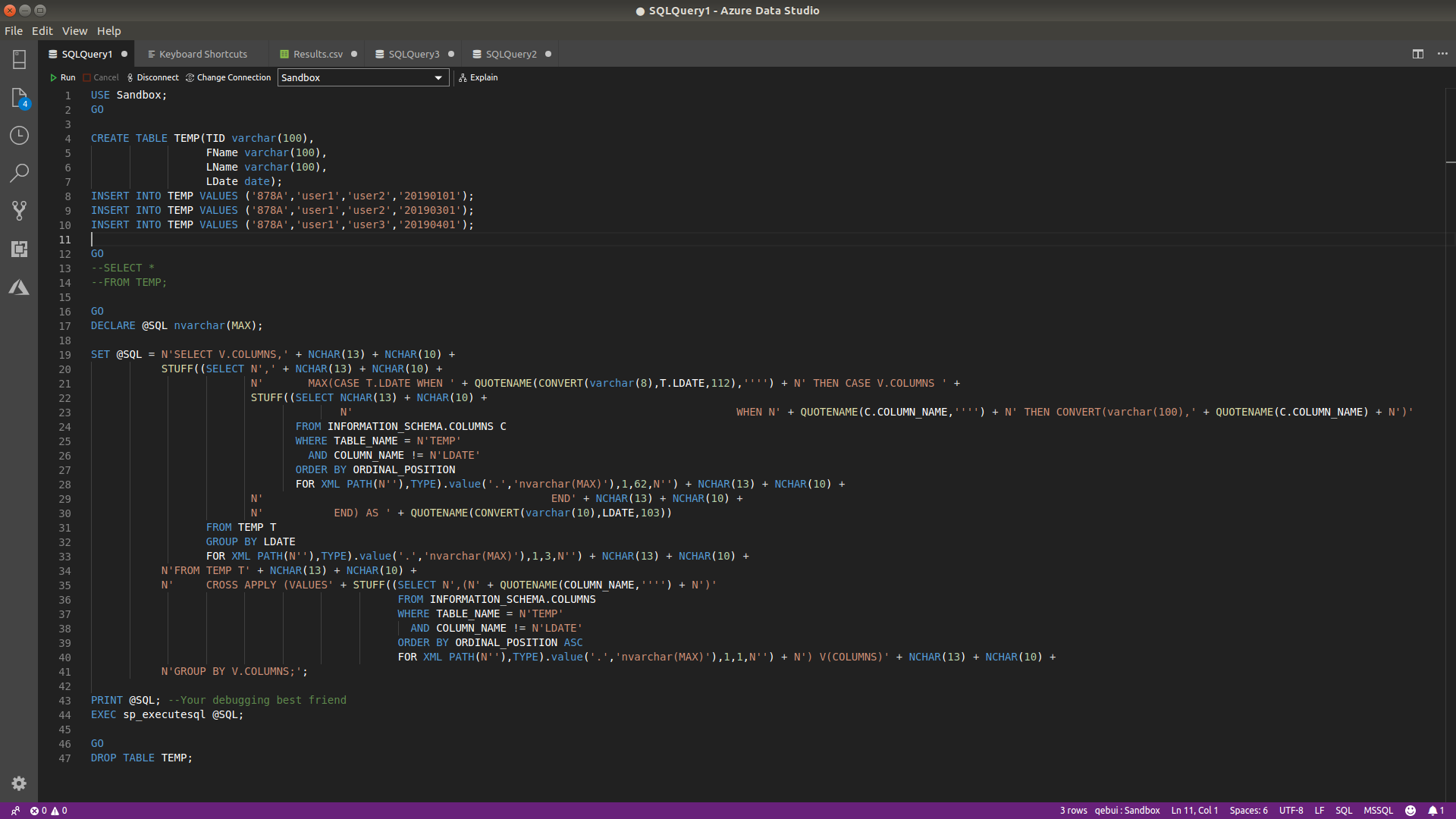# Wide code doesn't look good

• Thom A

SSC Guru

Points: 98207

The title says it all, but I pasted some SQL, which is pretty wide, and it looks really bad on the forums:

`USE Sandbox;GOCREATE TABLE TEMP(TID varchar(100),                  FName varchar(100),                  LName varchar(100),                  LDate date);INSERT INTO TEMP VALUES ('878A','user1','user2','20190101');INSERT INTO TEMP VALUES ('878A','user1','user2','20190301');INSERT INTO TEMP VALUES ('878A','user1','user3','20190401');GO--SELECT *--FROM TEMP;GODECLARE @SQL nvarchar(MAX);SET @SQL = N'SELECT V.COLUMNS,' + NCHAR(13) + NCHAR(10) +           STUFF((SELECT N',' + NCHAR(13) + NCHAR(10) +                         N'       MAX(CASE T.LDATE WHEN ' + QUOTENAME(CONVERT(varchar(8),T.LDATE,112),'''') + N' THEN CASE V.COLUMNS ' +                         STUFF((SELECT NCHAR(13) + NCHAR(10) +                                       N'                                                            WHEN N' + QUOTENAME(C.COLUMN_NAME,'''') + N' THEN CONVERT(varchar(100),' + QUOTENAME(C.COLUMN_NAME) + N')'                                FROM INFORMATION_SCHEMA.COLUMNS C                                WHERE TABLE_NAME = N'TEMP'                                  AND COLUMN_NAME != N'LDATE'                                ORDER BY ORDINAL_POSITION                                FOR XML PATH(N''),TYPE).value('.','nvarchar(MAX)'),1,62,N'') + NCHAR(13) + NCHAR(10) +                         N'                                             END' + NCHAR(13) + NCHAR(10) +                         N'           END) AS ' + QUOTENAME(CONVERT(varchar(10),LDATE,103))                  FROM TEMP T                  GROUP BY LDATE                  FOR XML PATH(N''),TYPE).value('.','nvarchar(MAX)'),1,3,N'') + NCHAR(13) + NCHAR(10) +           N'FROM TEMP T' + NCHAR(13) + NCHAR(10) +           N'     CROSS APPLY (VALUES' + STUFF((SELECT N',(N' + QUOTENAME(COLUMN_NAME,'''') + N')'                                                FROM INFORMATION_SCHEMA.COLUMNS                                                WHERE TABLE_NAME = N'TEMP'                                                  AND COLUMN_NAME != N'LDATE'                                                ORDER BY ORDINAL_POSITION ASC                                                FOR XML PATH(N''),TYPE).value('.','nvarchar(MAX)'),1,1,N'') + N') V(COLUMNS)' + NCHAR(13) + NCHAR(10) +           N'GROUP BY V.COLUMNS;';PRINT @SQL; --Your debugging best friendEXEC sp_executesql @SQL;GODROP TABLE TEMP;`

Conversely, in Azure Data Studio, it looks fine to read (attached image).Could the code windows have a horizontal scroll bar, if the area the posts use isn't going to get larger? Otherwise complex code like this really is an unreadable mess. It would be good if that is applied to the articles as well, if they "suffer" from the same problem.

Thom~

Excuse my typos and sometimes awful grammar. My fingers work faster than my brain does.

• Steve Jones - SSC Editor

SSC Guru

Points: 714600

Added this to the bug for styling. I'm torn on this, as sometimes really long code starts to break down in styling. If we can get a side scrolling section, that might be best.

• Ed Wagner

SSC Guru

Points: 286954

Agreed on the horizontal scrollbar.

Viewing 3 posts - 1 through 3 (of 3 total)